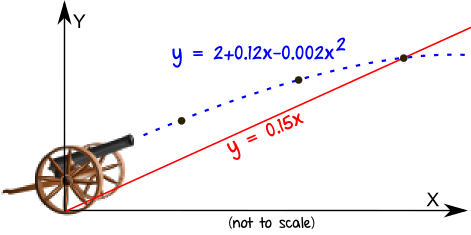## Trigonometry 1

Trigonometry 1: Applying trigonometric skills to solve equations

By the end of this topic you will be able to:
• convert Degrees to Radian measure and vice versa
• recall exact values in and calculate using radian measure
• translate graphs of trig functions
• solve trigonometric equations in degrees or radians

• including those involving the wave function or trigonometric formulae or identities, in a given interval

Homework: Trigonometry HW1

Due: Monday 20th December

ALL pupils should be able to Q1-4

MOST pupils should be able to complete Q5

SOME : Not applicable for HW1

DO NOT DO Q6

🙂

## The CircleNew Topic: The Circle

By the end of this topic you will:

• know that the equation of the circle centre (a,b) and radius r is (x – a)² + (y – b)² = r²

• know that x² + y² + 2gx + 2fy + c = 0 represents a circle centre (–g, –f) and
radius r = √(g² + f² – c), provided g² + f² – c > 0

• be able to determine the equation of a circle

• be able to solve problems with the intersection of a line and a circle,
and a tangent to a circle

• be able to determine whether two circles touch each other

Homework: Circles Set2

Due: Monday 7th December

ALL pupils should be able to complete Q1 – 4

MOST pupils should be able to complete Q7

SOME pupils should be able to complete Q6

DO NOT DO Q5

Circles Set2 Solutions

🙂

## DifferentiationNew Topic: Differentiation, MiA Higher Ch3

By the end of this topic you will:
• be familiar with the terminology associated with differentiation
• understand the principles of differentiation
• be able to derive the gradient formula of a given function
• be able to calculate the gradient of a tangent to a curve
• be able to use differentiation to solve optimisation problems

Homework 1: Differentiation 1 Set1

Due: Friday 3:20pm – submit to Mr H’s office

ALL pupils should be able to complete Q1 & 2

MOST pupils should be able to complete Q4

SOME pupils should be able to complete Q3

Homework 2: Differentiation HW2

Due: Wednesday 18th November

ALL pupils should be able to complete Q1 – 4

MOST pupils should be able to complete Q5 & 6

SOME pupils should be able to complete Q7

Differentiation HW2 – Solutions

🙂

## Composite FunctionsBy the end of this lesson you will:

• be able to find f(g(x)) given f(x) and g(x)

Homework: Composite and Inverse Functions Set1

Due: TBC

.

## Completing the Square Practice

Completing the Square worksheet for Friday 11/09/15

.

## Translating Functions

Desmos Calculator Activity

Explore the effects of changing the vales of a, b, c and d individually.

Discuss and agree the translations:

y = f(x + a)

y = f(x) + b

y = cf(x)

y = f(dx)

Click the graph below to start the activity:🙂

Click the image to view an explanation….## PolynomialsNew Topic: Polynomials

By the end of this topic you will be able to:
• Factorise a cubic polynomial expression with unitary x3 coefficient
• Solve cubic polynomial equations with unitary x3 coefficient
• Factorise a cubic or quartic polynomial expression where the term of highest degree has a non-unitary coefficient
• Solve a cubic or quartic polynomial equation, where the term of highest degree has a non-unitary coefficient
• Find the coordinates of the point(s) of intersection of a straight line and a curve or of two curves

Homework: Polynomials Set1 (Q1-6 only)

Due: Thursday 17th September

Please check the solutions below and attempt the equivalent questions from the Set 2 sheet to those you struggled with first time.  These should be handed in with your next homework or earlier. Please see me for help if necessary.

Polynomials Set 1 Solutions

Polynomials Set2

🙂By the end of this topic you will be able to:
• Given the nature of the roots of a quadratic equation, use the discriminant to find an unknown
• Finding the coordinates of the point(s) of intersection of a straight line and a curve or of two curves
• know condition for tangency; intersection of line and parabola (lines and curves)

Due: Wednesday 2nd September

Please check the solutions below and attempt the equivalent questions from the Set 2 sheet to those you struggled with first time.  These should be handed in with your next homework or earlier. Please see me for help if necessary.

🙂

## Euler Line

Euler Line – Group Investigation

In groups of 2 or 3:

Find the equation of the Euler line of the triangle with vertices A(-2, -4), B(2, 4) and C(12, -4).

Your group submission should be in the form of a presentation in a form of your choice. Your presentation must include:
• a fully worked solution with explanation
• accurate diagram
• other relevant information you discovered during your investigation

All members of your group should be prepared to present your findings to the class.

Due: Wednesday 30th September

Euler Line Investigation Worksheet